×
Get Full Access to SFSU - CHEM 101 - Study Guide - Midterm
Get Full Access to SFSU - CHEM 101 - Study Guide - Midterm

×

SFSU / Chemistry / CHEM 101 / How would the reaction rate be changed if more cl2 was added to the fo

# How would the reaction rate be changed if more cl2 was added to the fo Description

##### Description: Acids & Bases -rate -thermal energy -collision theory -conjugate pairs Strengths of Acids and Bases Authorization of H2O Buffer Solutions Functional Groups Biomolecules
10 Pages 136 Views 2 Unlocks
Reviews

Chemistry Study Guide: Acids & Bases

## How would the rate of reaction be changed if more cl2 was added to the following chemical 2 reaction?● The relationship between reaction rate and reactant concentration is that as reactant concentration increased, rate increases ; Directly Proportional.

Rate:​ the speed at which a chemical reaction proceeds.

Thermal​ ​energy​: speed of particles.

Directly proportional

More reactant

Faster rate

Inversely proportional

More reactant

Slower rate

No relationship

More reactant

Same rate

## How does increasing temperature increase reaction rate?● The relationship between reaction rate and reaction temperature is that as reaction temperature increases, reaction rate increases ; Directly proportional.

Directly proportional

Higher temperature

Higher rate

Inversely proportional

Higher temperature

Slower rate

No relationship

Higher temperature

Same rate

Example:

How would the rate of reaction be changed if more Cl was added to the following chemical 2 reaction?

## How does a catalyst effect collision geometry?2P (s) + 5Cl (g) 2P (s) It increases!! 2 → Cl5

Example:

How would the rate of reaction be changed if the temperature decreased? 2P (s) + 5Cl (g) 2P (s) It decreases!! 2 → Cl5If you want to learn more check out What is matlab data?

But… Why? How?

Collision​ ​Theory:​ Reactant molecules must collide in order for a reaction to occur. They must collide with sufficient energy and correct geometry.

Example:

How does increasing reactant concentration increase the rate?Increasing reactant concentration increases the frequency of collisions, and therefore increases reaction rate.

Example:

How does increasing temperature increase reaction rate?

● Increasing temperature, increasing speed of particles, and increases collision frequency. ● Increasing temperature, increases energy, with which reactants collide increasing likelihood of collision with sufficient energy. Don't forget about the age old question of What is the definition of the trouble functions?
We also discuss several other topics like What is the meaning of hcl in chemistry?

Example:

How would the rate of reaction be changed if a catalyst were added to the reactant mixture? 2P (s) + 5Cl (g) 2P (s) It increases!! 2 → Cl5

Example:

How does a catalyst effect collision geometry?

● Decreases Ea

● Provides a favorable collision geometry, decreases energy barrier.Acids & Bases:

An acid​ is a substance that donates H ions. (proton donor) + A base​ is a substance that accepts / takes H ions. (proton acceptor) + Don't forget about the age old question of What is the 305 ramac?

Conjugate​ ​Pairs​:

After an acid reacts (donates H ) it becomes its conjugate base. + After a base reacts (accepts H ) it becomes its conjugate acid. + Don't forget about the age old question of What is the formula for nernst equation?

Pairs are listen with acid first.

Acid / base differ by H+

Example:

H 2O / OH−

NH 4/ NH 3

NH 3/ NH 2−

Example:

What is the conjugate base of HCO ? 3−

HCO 3/ CO Example:

2−

3

What is the conjugate acid of HCO ? 3−

H 2CO 3/ HCO 3−

Strength​ ​of​ ​Acids:

+ H+ If you want to learn more check out Who is martin french?

● Strong Acids strongly donate H , 100% of acid molecules donate their (first) .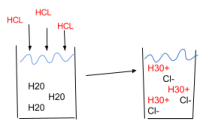HCl (aq) + H O (l) O (aq) Cl (aq) forward error indicates one strong reactant. 2 → H 3+ +−

● Weak Acids weakly donate H , usually only 1% of molecules donate , weak acids + H+ are not very reactive.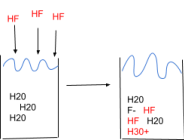HF (aq) + H O (l) O (aq) F (aq) double arrow indicated two weak reactants. 2 ↔ H 3+ +−

Strength​ ​of​ ​Bases:

● A strong base strongly accepts H+

● A weak base weakly accepts H+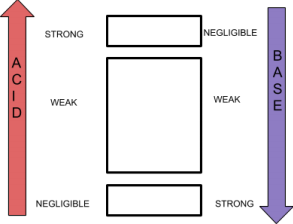Example:

What is the strength relationship between conjugate acid/base pairs?

The weaker an acid, the stronger its conjugate base (and so on).

Authorization​ ​of​ ​H2O:Example:

A solution has a [OH-] = 3 x 10^-2 M

This solution is…

Basic!!

The [OH-] is greater than neutral, 3 x 10^-2 M > 1 x 10^-7 M, therefore there is more OH- ; solution is basic.

Example:

A solution has a [OH-] = 3 x 10^-12 M

This solution is…

Acidic!!

[OH-] is less than 1 x 10^-7 M, thus there is less OH-, more H3O+ ; solution is acidic. Example:

A solution has a [H3O+] = 3 x 10^-12 M

This solution is…

Basic!!

[H30+] is less than 1 x 10^-7 M, thus there is less H30+ , more OH- ; solution is basic.

Buffer​ ​Solutions:

● Resistant to [H30+] / [OH-] changes, even when strong acid or strong base is added. ● Buffers must contain both a weak acid and weak base, conjugate pairs, in roughly equal amounts.

Skeletal​ ​Line​ ​Structures:

● Each end & each vertex represents a carbon atom.

● The line segments represent bonds

● The hydrogen bonded to carbon are implied

● All other atoms, including hydrogen bounds to other atoms, are expressly written.Functional​ ​Groups:

An atom or a set of atoms bonded in a very specific way.

Group

Name

R-O-H

● Alcohol

● Polar

● H-bond

R-O-R’

● Ether

● polar

R-NH x

● Amine

● Polar

● H-bond

● Acts as a base

Carbonyl​ ​Functional​ ​Groups​ ​(C=O)

Group

Name

R-C-O-H

||

O

Carboxylic acid

● Acts as acid

● Not an alcohol

R-C-O-R’

||

O

Ester

● Not an ether

R-C-NH x

||

O

Amide

● Not an amine

● Neutral (not acidic nor basic)

R-C-H

||

O

Aldehyde

● At the end

R-C-R’

||

O

Ketone

● In the middle

Example:

What is the functional group?Amine!! (NH2)

Example:

What is the functional group?

H3C-O-C

Ether!!!

Example:

What is the functional group?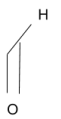Aldehyde!!

Example:

What is the functional group?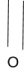Ketone!!

Biomolecules:

● Large

● Organic

● Complex structure

4 types:

- Proteins

- Carbohydrates

- Lipids

- Nucleic acids

Monomer+monomer+monomer, etc = polymer

● Examine the small unit (monomer)

- Look for structural patterns

● Bond many monomers together to make a polymer

- Look for structural patterns

**structure=function**

Proteins:

● The small “monomer” unit of proteins are amino acids.

● There are ~ 20 amino acids.

● All amino acids have a main structure, each one differs in its side chain “R” ● All amino acids are polar, all contain a basic amine group, and on acidic carboxylic acid group.

Polar (has functional group)

Nonpolar (just hydrocarbon)

Acidic

● Carboxylic acid

neutral

Basic

● amine

Neutral

● All others

-Proteins are polymers, also called polypeptides, that range in size but are usually composed of 50+ Amino Acids.

-Primary (1 degree) structure of a protein is the sequence of amino acids bonded together via peptide bonds.

-If we want to bond 2 Amino Acids together, we need to “make room” for new bonds.

Page ExpiredIt looks like your free minutes have expired! Lucky for you we have all the content you need, just sign up here